# Vibration of rectangle plate with variable density and Poisson’s ratio

## Amit Sharma1, Vijay Kumar2, Reeta Bhardwaj3

1, 2, 3Department of Mathematics, Amity University Haryana, Gurgaon, India

2Corresponding author

Vibroengineering PROCEDIA, Vol. 22, 2019, p. 68-73. https://doi.org/10.21595/vp.2019.20547
Received 17 January 2019; accepted 28 January 2019; published 15 March 2019

36th International Conference on Vibroengineering in Dubai, United Arab Emirates, March 15-17th, 2019

Copyright © 2019 Amit Sharma, et al. This is an open access article distributed under the Creative Commons Attribution License, which permits unrestricted use, distribution, and reproduction in any medium, provided the original work is properly cited.
Views 72
Abstract.

The effect of variation in density and Poisson’s ratio is examined, on the vibration of rectangle plate with linear variable thickness. Bi parabolic temperature effect on the plate is also studied and vibrational modes of rectangular plate is calculated by using Rayleigh Ritz method. Authors also compared the present finding with the published results.

Keywords: vibration, density, Poisson’s ratio, variation.

#### 1. Introduction

Isotropic rectangle plate is used in various engineering like civil, mechanical, automobile, aerospace and aircraft engineering. Due to it’s wide applications, it is required to know the vibration characteristics of isotropic rectangular plates. The plates made of non-homogeneous material along with variable thickness has significant impact rather than the homogeneous and uniform plates.

An excellent study related to plate vibration, on different edge conditions is given in [1-7]. A finite layer method  is used to developed three dimensional linear and small deformation solution for the vibration of rectangular plate on different boundary conditions. The mean square bending moment of a rectangular plate has been discussed in . Rayleigh Ritz technique is used to calculate vibrational frequency of non-homogeneous trapezoidal plate  on clamped and simply supported edge condition. The effect of rotary inertia  on vibration of rectangular plate with central cutout is studied, using first order shear deformation theory. Forced vibration of orthotropic circular plate with linear variation in thickness, resting on elastic foundation is discussed in . The effect of axial tension, viscosity coefficients and ratio of length-to-depth is studied on transverse vibration of viscoelastic Timoshenko beam columns . Vibration of square and skew plate [14, 15] is discussed with variation in thickness, density and temperature using Classical plate theory. The effect of circular variation in thickness and Poisson’s ratio on frequency of square plate is presented in . Vibration of circular plate with parabolic thickness, exponential variation in density and Young’s modulus is discussed in  using Ritz method. The effect of various plate parameters [18, 19] on vibration of skew and rectangular plate is presented, on clamped edge, using Rayleigh Ritz method. The temperature effect on vibration of rectangular plate made of non-homogeneous material, with variable thickness, is discussed in [20, 21] using Classical plate theory.

The present paper provides the effect of variation in density as well in Poisson’s ratio on frequency parameter of the plate. Authors also computed the effect of thickness and temperature on frequency. A comparison of frequency with  and , with respect to thermal gradient is also given, to validate the finding of the present study.

#### 2. Analysis

Consider a thin rectangular plate with length $a$, breadth $b$, thickness $l$, density $\rho$ and Poisson’s ratio $\nu$referred to cartesian coordinates ($\zeta$, $\psi$).

The maximum kinetic energy of the plate is given by:

(1)
${T}_{s}=\frac{1}{2}{\omega }^{2}{\int }_{0}^{a}\mathrm{‍}{\int }_{0}^{b}\mathrm{‍}\rho l{\mathrm{\Phi }}^{2}d\psi d\zeta ,$

where $\mathrm{\Phi }$ is deflection function and $\omega$ is natural frequency.

The maximum strain energy of the plate is given by:

(2)
${V}_{s}=\frac{1}{2}{\int }_{0}^{a}\mathrm{‍}{\int }_{0}^{b}\mathrm{‍}{D}_{1}\left[{\left(\frac{{\partial }^{2}\mathrm{\Phi }}{\partial {\zeta }^{2}}\right)}^{2}+{\left(\frac{{\partial }^{2}\mathrm{\Phi }}{\partial {\psi }^{2}}\right)}^{2}+2\nu \frac{{\partial }^{2}\mathrm{\Phi }}{\partial {\zeta }^{2}}\frac{{\partial }^{2}\mathrm{\Phi }}{\partial {\psi }^{2}}+2\left(1-\nu \right){\left(\frac{{\partial }^{2}\mathrm{\Phi }}{\partial \zeta \partial \psi }\right)}^{2}\right]d\psi d\zeta ,$

where ${D}_{1}=E{l}^{3}/12\left(1-{\nu }^{2}\right)$, here $E$ is Young’s modulus of the plate.

Rayleigh Ritz method requires:

(3)
$I=\delta \left({V}_{s}-{T}_{s}\right)=0.$

Using Eq. (1) and Eq. (2), we get:

(4)

Now introducing non-dimensional variable ${\zeta }_{1}=\zeta /a$, ${\psi }_{1}=\psi /a$ together with linear variation in thickness, circular variation in density and exponential variation in Poisson’s ratio:

(5)

where ${l}_{0}$, ${\rho }_{0}$ and ${\nu }_{0}$ are the thickness, density and Poisson’s ratio, respectively at origin. Also , and are taper constant and non-homogeneity parameters.

The temperature variation is parabolic on the plate and is given by:

(6)
$\tau ={\tau }_{0}\left(1-\frac{{\zeta }^{2}}{{a}^{2}}\right)\left(1-\frac{{\psi }^{2}}{{b}^{2}}\right),$

where $\tau$ and ${\tau }_{0}$ are the temperature on the plate at any point and at the origin respectively. The temperature dependent modulus of elasticity is:

(7)
$E={E}_{0}\left(1-\gamma \tau \right),$

where $\gamma$ is called slope of variation. Using Eq. (6), Eq. (7) becomes:

(8)
$E={E}_{0}\left[1-\alpha \left(1-\frac{{\zeta }^{2}}{{a}^{2}}\right)\left(1-\frac{{\psi }^{2}}{{b}^{2}}\right)\right],$

where is called temperature gradient. Using non-dimensional variable along with Eq. (5) and Eq. (8), the functional in Eq. (4) become:

(9)

where ${D}_{0}={E}_{0}{l}_{0}^{3}/\text{12}$ and ${\lambda }^{2}={\rho }_{0}{\omega }^{2}{l}_{0}{a}^{4}/{D}_{0}.$

Now assuming the deflection function as:

(10)
$\mathrm{\Phi }\left(\zeta ,\psi \right)=\left[{\left(\frac{\zeta }{a}\right)}^{p}{\left(\frac{\psi }{b}\right)}^{q}{\left(1-\frac{\zeta }{a}\right)}^{r}{\left(1-\frac{\psi }{b}\right)}^{s}\right]\left[\sum _{i=0}^{n}{\mathrm{\Omega }}_{i}{\left\{\left(\frac{\zeta }{a}\right)\left(\frac{\psi }{b}\right)\left(1-\frac{\zeta }{a}\right)\left(1-\frac{\psi }{b}\right)\right\}}^{i}\right],$

where ${\mathrm{\Omega }}_{1}$ and ${\mathrm{\Omega }}_{2}$ represents arbitrary constants and $p$, $q$, $r$. $s$ take values 0, 1 and 2 depending upon the support edge condition i.e., take value 0 for free edge, 1 value for simply supported and 2 value for clamped edge.

In order to minimize the functional given in Eq. (9), we require that:

(11)

After simplifying we get a homogenous system of equations in ${\mathrm{\Omega }}_{i}$ whose non-zero solution gives equation of frequency as:

(12)
$\left|C-{\lambda }^{2}D\right|=0,$

where $C=\left[{c}_{ij}\right]$ and $D=\left[{d}_{ij}\right]$ are square matrix of order ($n$ + 1) and $i=$ 0, 1, 2, ..., $n$ and $j=$ 0, 1, 2, ..., $n.$

#### 3. Numerical illustration and discussions

The frequency Eq. (12) is solved for first two modes of vibration on clamped edges for various values of temperature gradient $\alpha$, taper constant $\beta$ and non-homogeneity parameters ${m}_{1}$, ${m}_{2}$ and presented with the help of figures. The value of aspect ratio is taken as $a$/$b=$ 1.5.

Fig. 1 shows the effect of non-homogeneity ${m}_{2}$ on vibrational frequency for three set of taper constants $\beta =$ 0.2, 0.4, 0.6, thermal gradient $\alpha =$ 0.2, 0.4, 0.6 and non-homogeneity parameter ${m}_{1}=$ 0.2, 0.4, 0.6. It has been observed that on all set values, frequency parameter increases with the increasing value of non-homogeneity parameter ${m}_{2}$.

Fig. 2 shows the effect of another non-homogeneity parameter ${m}_{1}$ on vibration frequency of the plate on three set value of taper constant $\beta =$ 0.2, 0.4, 0.6, thermal gradient $\alpha =$ 0.2, 0.4, 0.6 and non-homogeneity parameter ${m}_{2}=$ 0.2, 0.4, 0.6. Here, increase in non-homogeneity ${m}_{1}$ results the decrease (opposite to the frequency presented in Fig. 1) in vibration frequecy, on all the set values.

Fig. 3 shows the effect of taper constant $\beta$ on vibration frequency of the plate on three set value of thermal gradient $\alpha =$ 0.2, 0.4, 0.6 and non-homogeneity parameters ${m}_{1}={m}_{2}=$ 0.2, 0.4, 0.6. It is seen that increase in taper constant results the increase in vibrational frequency, on all the three set values. Although the combined increase in mentioned plate parameters results the decrease in vibration frequency.

Fig. 4 depicts the behavior of frequency with respect to increasing value of thermal gradient $\alpha$ on three set value of taper constant $\beta =$ 0.2, 0.4, 0.6 and non-homogeneity parameters ${m}_{1}={m}_{2}=$ 0.2, 0.4, 0.6. Here, increase in thermal gradient results the decrease in vibrational frequency on all the three set values. Although the combined increase in mentioned plate parameters results the increase in frequency parameter.

Fig. 1. Non-homogeneity ${m}_{2}$ vs frequency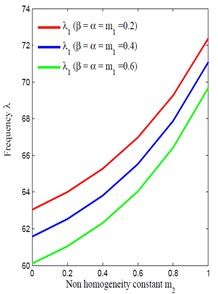a)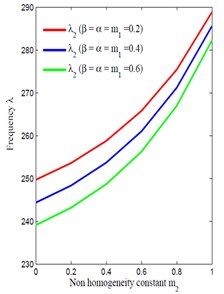b)

Fig. 2. Non-homogeneity ${m}_{1}$ vs frequency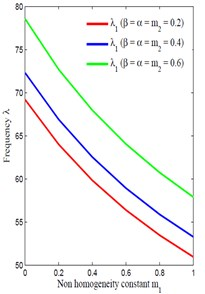a)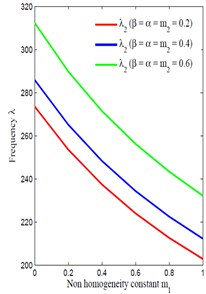b)

Fig. 3. Taper constant $\beta$ vs frequency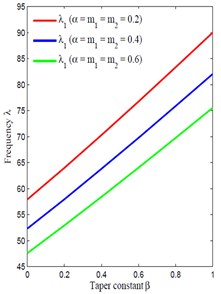a)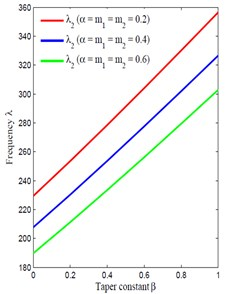b)

Fig. 4. Thermal gradient $\alpha$ vs frequency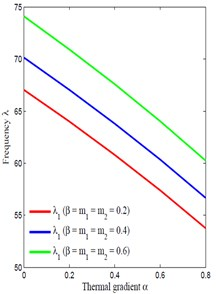a)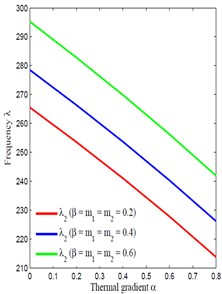b)

#### 4. Comparison of results

A comparison of frequency parameters of present paper has been made with  and  in Fig. 5.

Fig. 5 shows the comparison of frequency modes of present study with  and  with respect to temperature gradient $\alpha$ for fixed value of non-homogeneity constant ${m}_{1}$ i.e., ${m}_{1}=$ 0.0, for two cases ${m}_{2}=\beta =$ 0.0 and ${m}_{2}=$ 0.0, $\beta =$ 0.6. The frequecy modes of present study is less when compared to frequency modes presented in  and . At ${m}_{2}=\beta =$ 0.0 the frequency modes of present study,  and  are coincides. At ${m}_{2}=$ 0.0, $\beta =$ 0.6 the frequency modes of present study is concides with frequency modes reported in  and less in comparison to the frequency modes reported in .

Fig. 5. Comparison of frequency modes with  and  corresponding to thermal gradient $\alpha$ for fixed value of ${m}_{1}=$ 0.0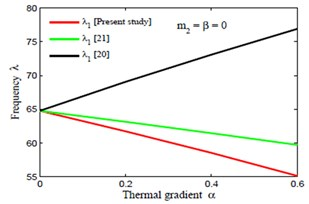a)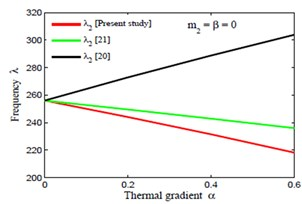b)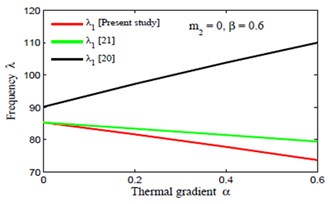c)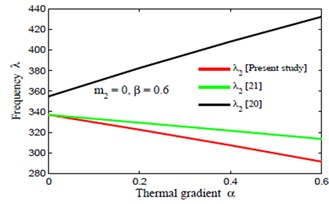d)

#### 5. Conclusions

From the results discussion and comparison, authors would like to record the followings conclusions:

1) The frequency in case of parabolic temperature variation (present study) is very less in comparison to exponential variation in temperature  and linear variation in temperature  as shown in Fig. 5.

2) Increase in non-homogeneity ${m}_{2}$ results the increase in frequency parameter while the increase in non-homogeneity ${m}_{1}$ results the decrease in frequency parameter. The rate of increase in frequency is high in comparison to rate of decrease in frequency.

3) The frequency parameters increasing with the increment in tapering parameter $\beta$ of the plate i.e., as thickness of the plate increasing, frequencies are also increasing as shown in Fig. 3.

4) The frequency parameter decreases with the increment in thermal gradient i.e., as the temperature on the plate increasing, frequencies are decreasing as shown in Fig. 4.

1. Leissa A. W. Vibration of Plates. Technical Report, sp-160, NASA, 1969. [Search CrossRef]
2. Leissa A. W. Recent research in plate vibrations: classical theory. The Shock and Vibration Digest, Vol. 9, Issue 10, 1977, p. 13-24. [Publisher]
3. Leissa A. W. Recent research in plate vibrations 1973-1976: complicating effects. The Shock and Vibration Digest, Vol. 10, Issue 12, 1978, p. 21-35. [Publisher]
4. Leissa A. W. Literature review: plate vibration research, 1976-1980: classical theory. The Shock and Vibration Digest, Vol. 13, Issue 9, 1981, p. 11-22. [Publisher]
5. Leissa A. W. Plate vibrations research 1976-1980: complicating effects. The Shock and Vibration Digest, Vol. 13, Issue 10, 1981, p. 19-36. [Publisher]
6. Leissa A. W. Recent studies in plate vibrations 1981-1985, part I: classical theory. The Shock and Vibration Digest, Vol. 19, Issue 2, 1987, p. 11-18. [Publisher]
7. Leissa A. W. Recent studies in plate vibrations 1981-1985, part II: complicating effects. The Shock and Vibration Digest, Vol. 19, Issue 3, 1987, p. 10-24. [Publisher]
8. Cheung Y. K., Chakrabarti S. Free vibration of thick, layered rectangular plates by a finite layer method. Journal of Sound and Vibration, Vol. 21, Issue 3, 1972, p. 277-284. [Publisher]
9. Chonan S. Random vibration of an initially stressed thick plate on an elastic foundation. Journal of Sound and Vibration, Vol. 71, Issue 1, 1980, p. 117-127. [Publisher]
10. Gupta A. K., Pragati S. Vibration study of non-homogeneous trapezoidal plates of variable thickness under thermal gradient. Journal of Vibration and Control, Vol. 22, Issue 5, 2016, p. 1369-1379. [Publisher]
11. Kalita K., Haldar S. Free vibration analysis of rectangular plates with central cutout. Cogent Engineering, Vol. 3, Issue 1, 2016, https://doi.org/10.1080/23311916.2016.1163781. [Publisher]
12. Ansari A. H. Forced response of polar orthotropic tapered circular plates resting on elastic foundation. Advances in Acoustics and Vibration, Vol. 2016, 2016, https://doi.org/10.1155/2016/1492051. [Publisher]
13. Chen L. Q., Peng L., Zhang A. Q., Ding H. Transverse vibration of viscoelastic Timoshenko beam-columns. Journal of Vibration and Control, Vol. 23, Issue 10, 2017, p. 1572-1584. [Publisher]
14. Sharma A. Thermal induced vibration of non-homogeneous tapered square plate. Journal of Engineering and Applied Sciences, Vol. 13, Issue 1, 2018, p. 2346-2351. [Search CrossRef]
15. Sharma A. Natural vibration of parallelogram plate with circular variation in density. Acta Technica, Vol. 63, Issue 6, 2018, p. 763-774. [Search CrossRef]
16. Sharma A., Kumar V., Raghav A. K. Vibrational frequency of circular tapered square plate. Romanian Journal of Acoustics and Vibration, Vol. 14, Issue 1, 2017, p. 21-27. [Search CrossRef]
17. Sharma S., Lal R., Singh N. Effect of non-homogeneity on asymmetric vibrations of non-uniform circular plates. Journal of Vibration and Control, Vol. 23, Issue 10, 2017, p. 1635-1644. [Publisher]
18. Sharma A. Vibration of skew plate with circular variation in thickness and Poisson’s ratio. Mechanics and Mechanical Engineering, Vol. 22, Issue 1, 2018, p. 43-52. [Publisher]
19. Sharma A., Raghav A. K., Kumar V., Sharma A. K. Effect of circular variation in thickness and linear variation in density on vibrational frequency. Progress in Advanced Computing and Intelligent Engineering, Advances in Intelligent System and Computing, Vol. 564, 2018, p. 501-509. [Publisher]
20. Khanna A., Kaur N. Effect of non-homogeneity on free vibration of visco-elastic rectangular plate with varying structural parameters. Journal of Vibroengineering, Vol. 15, Issue 4, 2013, p. 2146-2155. [Search CrossRef]
21. Khanna A., Kaur N. A study on vibration of tapered rectangular plate under non-uniform temperature field. Mechanika, Vol. 20, Issue 4, 2014, p. 376-381. [Publisher]

#### Cited By

 Journal of Vibration and Control Reeta Bhardwaj, Naveen Mani, Amit Sharma 2020